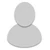5,359 Pages

• The champion statboxes round all their values up to the third or second decimal.

Many values show many decimals because of riot's growth formula base + growth × (lvl − 1) × (0.7025 + 0.0175 × (lvl − 1)) and i agree these should be rounded up in the statbox.

However i don't agree that it also rounds the base value (aka the lvl 1 value), which is the value written inside the champion data templates, and the lvl 18 value. At lvl 18 the growth formula above becomes base + growth × 17, so there's no extra decimal number.

My suggestion is to not round base values (which many people use to calculate stuff) and values at lvl 18 in the champion statboxes. Since this is a global suggestion i wanted to open a discussion on it.

•